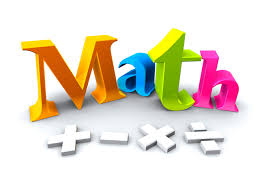MathematicsThroughout the year the following topics will be explored.

• Number Sense and Place Value
• Estimation
• Patterns and Algebra
• Operations (Addition, Subtraction, Multiplication, and Division)
• Measurement
• Money
• Geometry
• Problem Solving
• Telling Time
• Fractions
• Data Analysis
• Graphing
• Probability